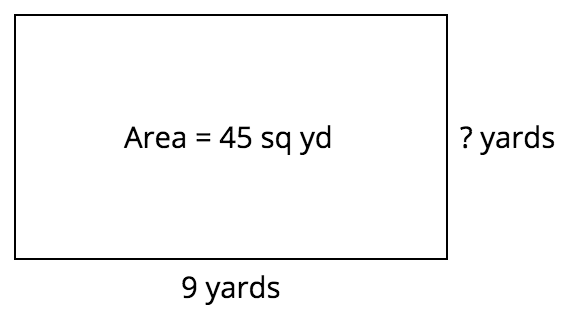# Area

## Objective

Find the area of a rectangle through multiplication of the side lengths.

## Common Core Standards

### Core Standards

?

• 3.MD.C.6 — Measure areas by counting unit squares (square cm, square m, square in, square ft, and improvised units).

• 3.MD.C.7.A — Find the area of a rectangle with whole-number side lengths by tiling it, and show that the area is the same as would be found by multiplying the side lengths.

?

• 2.G.A.2

• 2.MD.A.1

## Criteria for Success

?

1. Find the area of a rectangle shown pictorially with labeled dimensions by multiplying its length and width, explaining how that is the same as counting individual units (MP.3, MP.7).
2. Find the side length of a rectangle shown pictorially with labeled dimensions given its area and one side length using the relationship between multiplication and division.

## Tips for Teachers

?

As a supplement to the Problem Set, students can play “Raging Rectangles,” from Building Conceptual Understanding and Fluency Through Games (Grade 3) by the North Carolina Department of Public Instruction.

### Remote Learning Guidance

If you need to adapt or shorten this lesson for remote learning, we suggest prioritizing Anchor Task 1 (benefits from worked example). Find more guidance on adapting our math curriculum for remote learning here.

#### Fishtank Plus

• Problem Set
• Student Handout Editor
• Vocabulary Package

?

### Problem 1

What is the area of the shape below?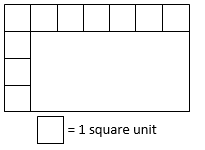Show or explain how you know.

### Problem 2

1. Find the area of the following rectangle.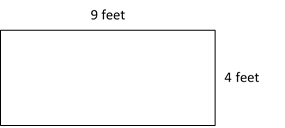1. Frederick draws another rectangle with the same area. What might the length and width of Frederick's rectangle be?

### Problem 3

1. The area of the rectangle below is 42 square feet. Find the missing side length.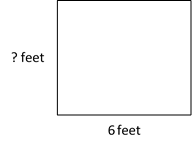1. Explain how you can use either multiplication or division to solve Part (a).

## Problem Set & Homework

### Discussion of Problem Set

• In what way is the area of #2(b) related to the area of #2(a)? How could you use the side lengths to help you figure out that 8 x 7 is double 4 x 7?
• Which shape in #2 is a square? How do you know?
• How are the rectangles in #2(a) and #5(c) similar? How are they different?
• Was anyone able to tell which two tables in #7 had the same area before multiplying them? How was that possible?
• Why is there a connection between a rectangle’s side lengths and its area?

?

### Problem 1

A rectangle has the measurements shown. Select the three ways to calculate the area of the rectangle in square centimeters.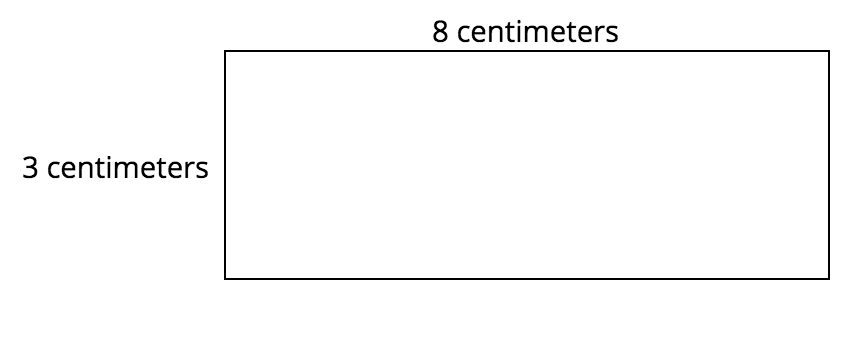### Problem 2

Find the side length of the rectangle below.